Question

# Let L be any non-empty language over an alphabet Σ. Show that L^2 ⊆ L^3 if...

Let L be any non-empty language over an alphabet Σ. Show that L^2 ⊆ L^3 if and only if λ ∈ L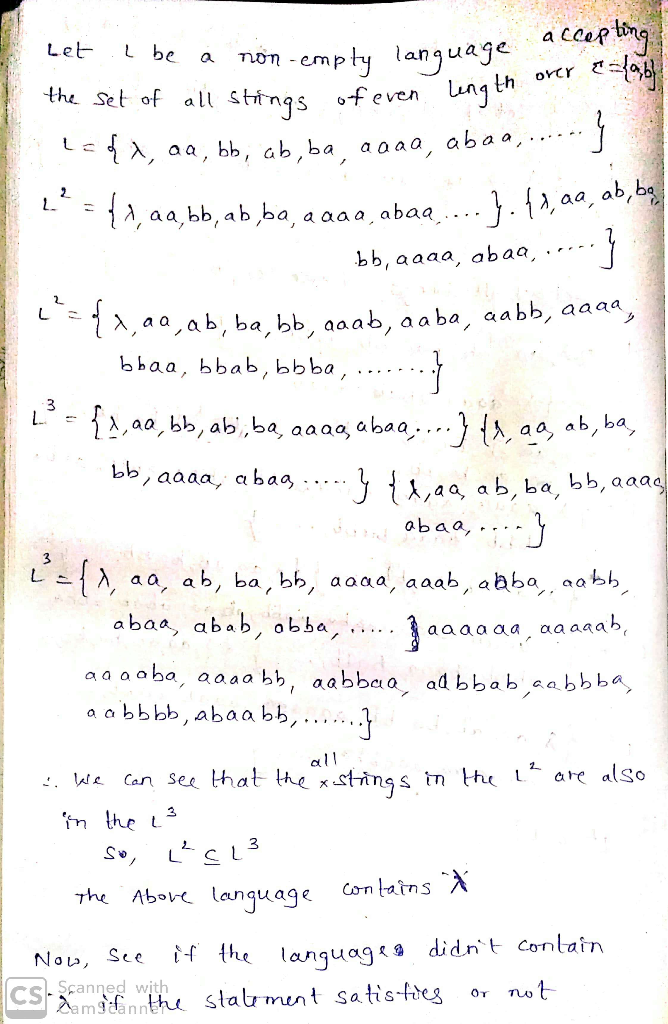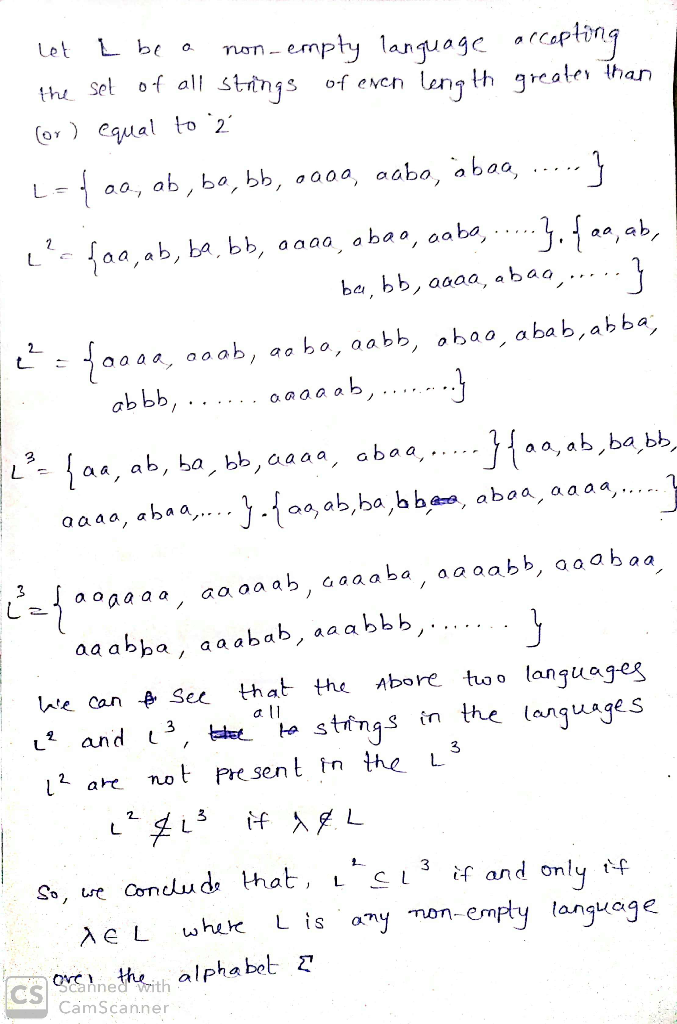#### Earn Coins

Coins can be redeemed for fabulous gifts.

Similar Homework Help Questions
• ### Clarity and precision (explanation) please 1. [5 Points) Let L be any non-empty language over an...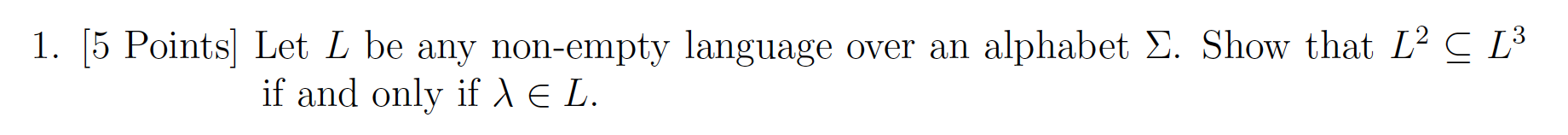Clarity and precision (explanation) please 1. [5 Points) Let L be any non-empty language over an alphabet E. Show that L2 C L if and only if le L.

• ### Consider the language defined over the alphabet Σ (0, 1):  2nin i. Show that L1...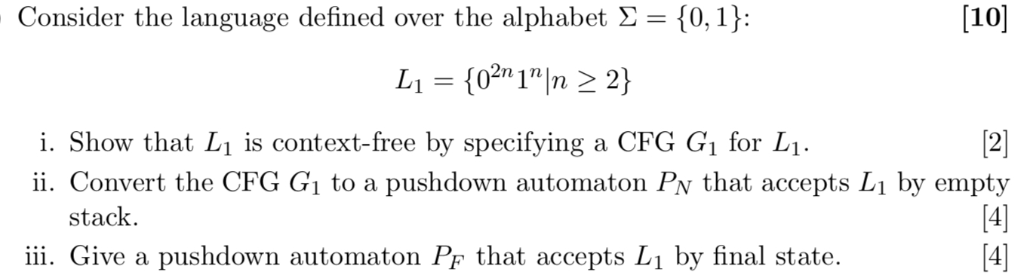Consider the language defined over the alphabet Σ (0, 1):  2nin i. Show that L1 is context-free by specifying a CFG Gi for L1 ii. Convert the CFG Gi to a pushdown automaton Pv that accepts L1 by empty 12 stack iii. Give a pushdown automaton PF that accepts L by final state

• ### Find a regular expression for the following language over the alphabet Σ = {a,b}. L = {strings th...

Find a regular expression for the following language over the alphabet Σ = {a,b}. L = {strings that begin and end with a and contain bb}.

• ### Let y-and Г be two alphabets, and let Г be the alphabet be the alphabet of vectors where the first element is from Σ and the second is from「 For example, if -(a,b) and Г-{0.1} then and B c Г be any...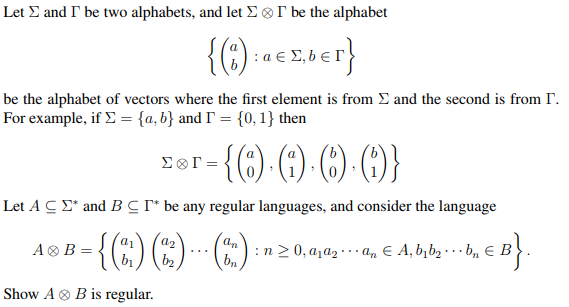Let y-and Г be two alphabets, and let Г be the alphabet be the alphabet of vectors where the first element is from Σ and the second is from「 For example, if -(a,b) and Г-{0.1} then and B c Г be any regular languages, and consider the language Let A Show A B is regular. Let y-and Г be two alphabets, and let Г be the alphabet be the alphabet of vectors where the first element is from Σ and...

• ### (d) Let L be any regular language. Use the Pumping Lemma to show that In >...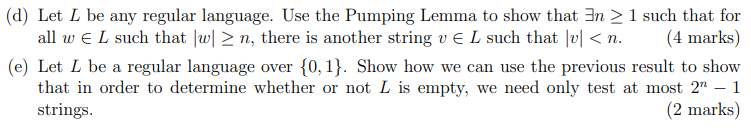(d) Let L be any regular language. Use the Pumping Lemma to show that In > 1 such that for all w E L such that|> n, there is another string ve L such that lvl <n. (4 marks) (e) Let L be a regular language over {0,1}. Show how we can use the previous result to show that in order to determine whether or not L is empty, we need only test at most 2" – 1 strings. (2...

• ### Question 1. Let Σ = {a, b}, and consider the language L = {w ∈ Σ...

Question 1. Let Σ = {a, b}, and consider the language L = {w ∈ Σ ∗ : w contains at least one b and an even number of a’s}. Draw a graph representing a DFA (not NFA) that accepts this language. Question 2. Let L be the language given below. L = {a n b 2n : n ≥ 0} = {λ, abb, aabbbb, aaabbbbbb, . . .} Find production rules for a grammar that generates L.

• ### John Doe claims that the language L, of all strings over the alphabet Σ = {...

John Doe claims that the language L, of all strings over the alphabet Σ = { a, b } that contain an even number of occurrences of the letter ‘a’, is not a regular language. He offers the following “pumping lemma proof”. Explain what is wrong with the “proof” given below. “Pumping Lemma Proof” We assume that L is regular. Then, according to the pumping lemma, every long string in L (of length m or more) must be “pumpable”. We...

• ### Specify a Turing machine with input alphabet Σ = {a, b} that recognizes the language L...

Specify a Turing machine with input alphabet Σ = {a, b} that recognizes the language L = { ww | w ∈ Σ ∗}. Is L decidable?

• ### Consider the following language on the alphabet Σ = (a L = {aaab, aab) Describe the...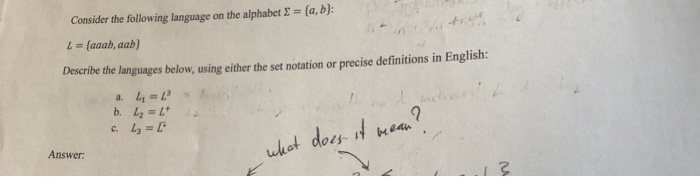Consider the following language on the alphabet Σ = (a L = {aaab, aab) Describe the languages below, using either the set notation or precise definitions in English: As whot does ea uk Answer

• ### Construct a Turing Machine (TM) that accepts the following language, defined over the alphabet Σ =...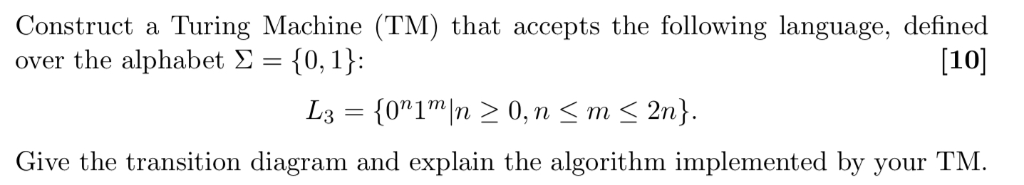Construct a Turing Machine (TM) that accepts the following language, defined over the alphabet Σ = {0,1): at accepts the tollowing language, define  Give the transition diagram and explain the algorithm implemented by your TM.